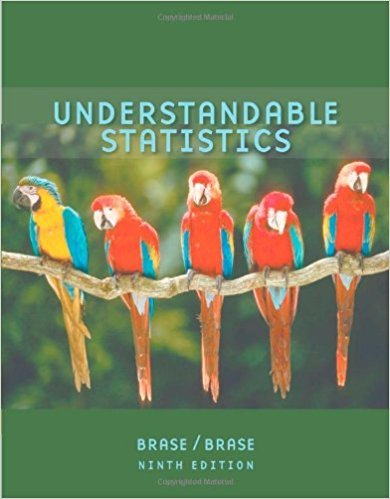×
×

# Solutions for Chapter 2.3: Organizing Data## Full solutions for Understandable Statistics | 9th Edition

ISBN: 9780618949922Solutions for Chapter 2.3: Organizing Data

Solutions for Chapter 2.3
4 5 0 318 Reviews
15
0
##### ISBN: 9780618949922

This textbook survival guide was created for the textbook: Understandable Statistics, edition: 9. Since 10 problems in chapter 2.3: Organizing Data have been answered, more than 35655 students have viewed full step-by-step solutions from this chapter. Understandable Statistics was written by and is associated to the ISBN: 9780618949922. Chapter 2.3: Organizing Data includes 10 full step-by-step solutions. This expansive textbook survival guide covers the following chapters and their solutions.

Key Statistics Terms and definitions covered in this textbook
• Analytic study

A study in which a sample from a population is used to make inference to a future population. Stability needs to be assumed. See Enumerative study

• Bias

An effect that systematically distorts a statistical result or estimate, preventing it from representing the true quantity of interest.

• Binomial random variable

A discrete random variable that equals the number of successes in a ixed number of Bernoulli trials.

• Causal variable

When y fx = ( ) and y is considered to be caused by x, x is sometimes called a causal variable

• Central limit theorem

The simplest form of the central limit theorem states that the sum of n independently distributed random variables will tend to be normally distributed as n becomes large. It is a necessary and suficient condition that none of the variances of the individual random variables are large in comparison to their sum. There are more general forms of the central theorem that allow ininite variances and correlated random variables, and there is a multivariate version of the theorem.

• Conditional variance.

The variance of the conditional probability distribution of a random variable.

• Continuity correction.

A correction factor used to improve the approximation to binomial probabilities from a normal distribution.

• Continuous random variable.

A random variable with an interval (either inite or ininite) of real numbers for its range.

• Convolution

A method to derive the probability density function of the sum of two independent random variables from an integral (or sum) of probability density (or mass) functions.

• Correction factor

A term used for the quantity ( / )( ) 1 1 2 n xi i n ? = that is subtracted from xi i n 2 ? =1 to give the corrected sum of squares deined as (/ ) ( ) 1 1 2 n xx i x i n ? = i ? . The correction factor can also be written as nx 2 .

• Correlation

In the most general usage, a measure of the interdependence among data. The concept may include more than two variables. The term is most commonly used in a narrow sense to express the relationship between quantitative variables or ranks.

• Defects-per-unit control chart

See U chart

• Dependent variable

The response variable in regression or a designed experiment.

• Discrete uniform random variable

A discrete random variable with a inite range and constant probability mass function.

• Empirical model

A model to relate a response to one or more regressors or factors that is developed from data obtained from the system.

• Estimate (or point estimate)

The numerical value of a point estimator.

• Expected value

The expected value of a random variable X is its long-term average or mean value. In the continuous case, the expected value of X is E X xf x dx ( ) = ?? ( ) ? ? where f ( ) x is the density function of the random variable X.

• False alarm

A signal from a control chart when no assignable causes are present

• Frequency distribution

An arrangement of the frequencies of observations in a sample or population according to the values that the observations take on

• Gaussian distribution

Another name for the normal distribution, based on the strong connection of Karl F. Gauss to the normal distribution; often used in physics and electrical engineering applications

×